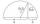# Square root + geometric average - math problems

#### Number of problems found: 7

• Geometric meanCalculate the geometric mean of numbers a=15.2 and b=25.6. Determine the mean by construction where a and b are the length of the lines.
• Annual growthThe population has grown from 25,000 to 33,600 in 10 years. Calculate what was the average annual population growth in%?
• Annual increaseThe number of cars produced increased from 45,000 to 47,000 in 3 years. Calculate the average annual increase in cars in%.
• Area of RTIn the right triangle has orthogonal projections of legs to the hypotenuse lengths 7 cm and 12 cm. Determine the area of ​​this triangle.
• Area of RTCalculate the right triangle area that hypotenuse has length 14, and one hypotenuse segment has length 5.
• Profit growthThe profit of a company increased by 25% during the year 1992, increased by 40% during the year 1993, decreased by 20% in the year 1994 and increased by 10% during the year 1995. Find the average growth in the profit level over the four years periods?
• InflationOnce upon a time, tsar owned a money printer and printed and printed. The result of printing money prices went up,in the first year 3.9 %, in the second 6%, in the third 4.7% and in the fourth 5.5%. Then tsar was failed in election. Calculate the average

We apologize, but in this category are not a lot of examples.
Do you have an interesting mathematical word problem that you can't solve it? Submit a math problem, and we can try to solve it.

We will send a solution to your e-mail address. Solved examples are also published here. Please enter the e-mail correctly and check whether you don't have a full mailbox.

Please do not submit problems from current active competitions such as Mathematical Olympiad, correspondence seminars etc...

Square root - math word problems. Geometric average - math word problems.